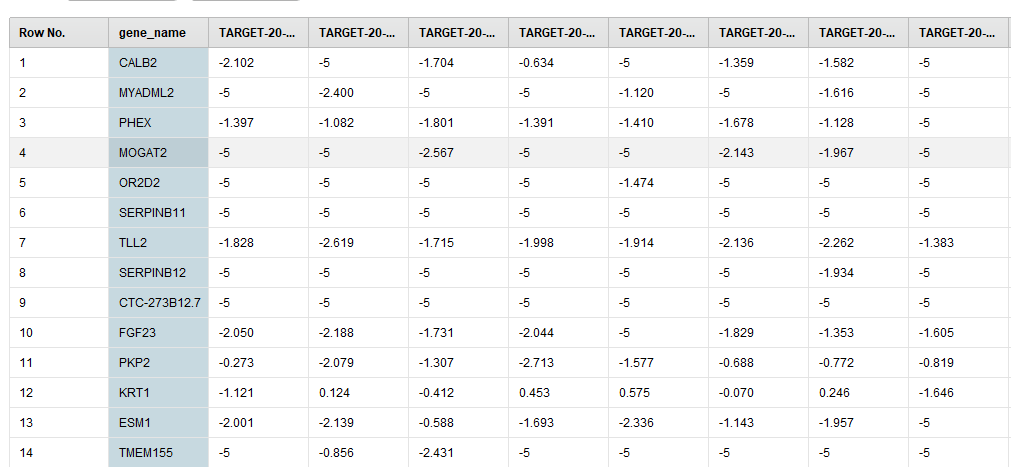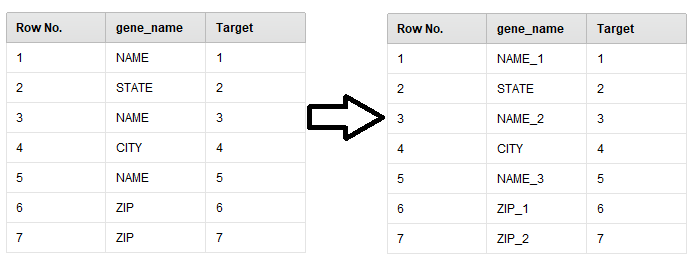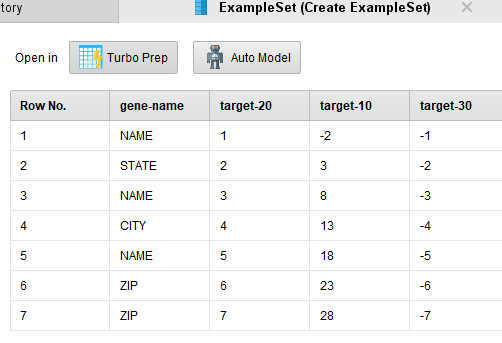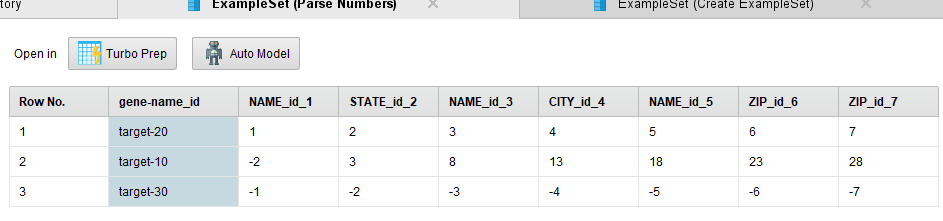# Remove Duplicate Examples

Member Posts: 9Contributor I
Hi,

I'm working on some genetics data. I have 6151 examples and 157 attributes. My attributes are patient IDs and my examples are gene names. My goal is to transpose the matrix table. Here is a sample of my data set:My problem now is I can't use the "Transpose" operator because there are duplicate row/example names. In order to transpose it, the attribute name needs to be unique. I wish to find all the pairs that have the same example names and edit their names. I was thinking about doing a loop, but I don't really know where to start and what operators to use to change the row names. Can somebody give me some advises on how to achieve this?

Thank you!

• Posts: 934Unicorn
Solution Accepted
Hi @gracewei,

Nice challenge, but honestly, I don't see any solution to perform automatically what you want to do with RapidMiner's native operator(s)...
... however there is a (relativ) simple solution using a Python script to perform this task.
Basically, the script add a number to the name of the duplicate and this number is incremented according to the number of duplicate(s) of a name.
Concretely the output example set looks like that :After executing this process, all the names/values of the "gene_name" attribute are unique et thus you can transpose your exampleset...

To execute this process, you need to :
- Install Python on your computer
- Install the Python Scripting extension in RapidMiner (from the Marketplace)

The process :
```<?xml version="1.0" encoding="UTF-8"?><process version="9.4.000-BETA">
<context>
<input/>
<output/>
<macros/>
</context>
<operator activated="true" class="process" compatibility="9.4.000-BETA" expanded="true" name="Process">
<parameter key="logverbosity" value="init"/>
<parameter key="random_seed" value="2001"/>
<parameter key="send_mail" value="never"/>
<parameter key="process_duration_for_mail" value="30"/>
<parameter key="encoding" value="SYSTEM"/>
<process expanded="true">
<operator activated="true" breakpoints="after" class="read_excel" compatibility="9.4.000-BETA" expanded="true" height="68" name="Read Excel" width="90" x="45" y="34">
<parameter key="excel_file" value="D:\Lionel\Formations_DataScience\Rapidminer\Tests_Rapidminer\Rename_Duplicates\Rename_Duplicates.xlsx"/>
<parameter key="sheet_selection" value="sheet number"/>
<parameter key="sheet_number" value="1"/>
<parameter key="imported_cell_range" value="A1"/>
<parameter key="encoding" value="SYSTEM"/>
<parameter key="first_row_as_names" value="true"/>
<list key="annotations"/>
<parameter key="date_format" value=""/>
<parameter key="time_zone" value="SYSTEM"/>
<parameter key="locale" value="English (United States)"/>
<list key="data_set_meta_data_information">
<parameter key="0" value="gene_name.true.polynominal.attribute"/>
<parameter key="1" value="Target.true.integer.attribute"/>
</list>
<parameter key="datamanagement" value="double_array"/>
<parameter key="data_management" value="auto"/>
</operator>
<operator activated="true" class="python_scripting:execute_python" compatibility="9.3.000" expanded="true" height="103" name="Execute Python" width="90" x="313" y="34">
<parameter key="script" value="import pandas&#10;from collections import Counter # Counter counts the number of occurrences of each item&#10;from itertools import tee, count&#10;&#10;# rm_main is a mandatory function, &#10;# the number of arguments has to be the number of input ports (can be none)&#10;def uniquify(seq, suffs = count(1)):&#10;    &quot;&quot;&quot;Make all the items unique by adding a suffix (1, 2, etc).&#10;&#10;    `seq` is mutable sequence of strings.&#10;    `suffs` is an optional alternative suffix iterable.&#10;    &quot;&quot;&quot;&#10;    not_unique = [k for k,v in Counter(seq).items() if v&gt;1] # so we have: ['name', 'zip']&#10;    # suffix generator dict - e.g., {'name': &lt;my_gen&gt;, 'zip': &lt;my_gen&gt;}&#10;    suff_gens = dict(zip(not_unique, tee(suffs, len(not_unique))))  &#10;    for idx,s in enumerate(seq):&#10;        try:&#10;            suffix = str(next(suff_gens[s]))&#10;        except KeyError:&#10;            # s was unique&#10;            continue&#10;        else:&#10;            seq[idx] += suffix&#10;&#10;def rm_main(data):&#10;&#10;  mylist = data['gene_name']            &#10;  uniquify(mylist, (f'_{x!s}' for x in range(1, 100)))&#10;  data['gene_name']  = mylist&#10;&#10;    # connect 2 output ports to see the results&#10;  return data"/>
<parameter key="notebook_cell_tag_filter" value=""/>
<parameter key="use_default_python" value="true"/>
<parameter key="package_manager" value="conda (anaconda)"/>
</operator>
<connect from_op="Read Excel" from_port="output" to_op="Execute Python" to_port="input 1"/>
<connect from_op="Execute Python" from_port="output 1" to_port="result 1"/>
<portSpacing port="source_input 1" spacing="0"/>
<portSpacing port="sink_result 1" spacing="0"/>
<portSpacing port="sink_result 2" spacing="0"/>
</process>
</operator>
</process>
```
Hope this will help in the future ...

Regards,

Lionel

• Member Posts: 9Contributor I
I just solved this by manually renaming the 11 duplicates. However, I'm still curious how this can be achieved using the operators!
• Administrator, Employee, RapidMiner Certified Analyst, RapidMiner Certified Expert, Member Posts: 239RM Data Scientist
Hi @gracewei,

You can use rapidminer operators to transpose and rename. But the tricky part is to create a new list of name without duplicates

```<?xml version="1.0" encoding="UTF-8"?><process version="9.3.001">
<context>
<input/>
<output/>
<macros/>
</context>
<operator activated="true" class="process" compatibility="9.3.001" expanded="true" name="Process">
<parameter key="logverbosity" value="init"/>
<parameter key="random_seed" value="2001"/>
<parameter key="send_mail" value="never"/>
<parameter key="process_duration_for_mail" value="30"/>
<parameter key="encoding" value="SYSTEM"/>
<process expanded="true">
<operator activated="true" class="utility:create_exampleset" compatibility="9.3.001" expanded="true" height="68" name="Create ExampleSet" width="90" x="45" y="85">
<parameter key="generator_type" value="comma separated text"/>
<parameter key="number_of_examples" value="100"/>
<parameter key="use_stepsize" value="false"/>
<list key="function_descriptions"/>
<list key="numeric_series_configuration"/>
<list key="date_series_configuration"/>
<list key="date_series_configuration (interval)"/>
<parameter key="date_format" value="yyyy-MM-dd HH:mm:ss"/>
<parameter key="time_zone" value="SYSTEM"/>
<parameter key="input_csv_text" value="gene-name,target-20,target-10,target-30&#10;NAME,&#9;1.0,&#9;-2.0,&#9;-1.0&#10;STATE,&#9;2.0,&#9;3.0,&#9;-2.0&#10;NAME,&#9;3.0,&#9;8.0,&#9;-3.0&#10;CITY,&#9;4.0,&#9;13.0,&#9;-4.0&#10;NAME,&#9;5.0,&#9;18.0,&#9;-5.0&#10;ZIP,&#9;6.0,&#9;23.0,&#9;-6.0&#10;ZIP,&#9;7.0,&#9;28.0,&#9;-7.0"/>
<parameter key="column_separator" value=","/>
<parameter key="parse_all_as_nominal" value="false"/>
<parameter key="decimal_point_character" value="."/>
<parameter key="trim_attribute_names" value="true"/>
</operator>
<operator activated="true" class="set_role" compatibility="9.3.001" expanded="true" height="82" name="Set Role" width="90" x="179" y="85">
<parameter key="attribute_name" value="gene-name"/>
<parameter key="target_role" value="regular"/>
</operator>
<operator activated="true" class="generate_id" compatibility="9.3.001" expanded="true" height="82" name="Generate ID" width="90" x="313" y="85">
<parameter key="create_nominal_ids" value="true"/>
<parameter key="offset" value="0"/>
</operator>
<operator activated="true" breakpoints="after" class="generate_concatenation" compatibility="9.3.001" expanded="true" height="82" name="Generate Concatenation" width="90" x="447" y="85">
<parameter key="first_attribute" value="gene-name"/>
<parameter key="second_attribute" value="id"/>
<parameter key="separator" value="_"/>
<parameter key="trim_values" value="true"/>
</operator>
<operator activated="true" breakpoints="after" class="transpose" compatibility="9.3.001" expanded="true" height="82" name="Transpose" width="90" x="581" y="85"/>
<operator activated="true" breakpoints="after" class="rename_by_example_values" compatibility="9.3.001" expanded="true" height="82" name="Rename by Example Values" width="90" x="715" y="85">
<parameter key="row_number" value="5"/>
</operator>
<operator activated="true" class="filter_example_range" compatibility="9.3.001" expanded="true" height="82" name="Filter Example Range" width="90" x="849" y="85">
<parameter key="first_example" value="4"/>
<parameter key="last_example" value="4"/>
<parameter key="invert_filter" value="true"/>
</operator>
<operator activated="true" class="parse_numbers" compatibility="9.3.001" expanded="true" height="82" name="Parse Numbers" width="90" x="983" y="85">
<parameter key="attribute_filter_type" value="all"/>
<parameter key="attribute" value=""/>
<parameter key="attributes" value=""/>
<parameter key="use_except_expression" value="false"/>
<parameter key="value_type" value="nominal"/>
<parameter key="use_value_type_exception" value="false"/>
<parameter key="except_value_type" value="file_path"/>
<parameter key="block_type" value="single_value"/>
<parameter key="use_block_type_exception" value="false"/>
<parameter key="except_block_type" value="single_value"/>
<parameter key="invert_selection" value="false"/>
<parameter key="include_special_attributes" value="false"/>
<parameter key="decimal_character" value="."/>
<parameter key="grouped_digits" value="false"/>
<parameter key="grouping_character" value=","/>
<parameter key="infinity_representation" value=""/>
<parameter key="unparsable_value_handling" value="fail"/>
</operator>
<connect from_op="Create ExampleSet" from_port="output" to_op="Set Role" to_port="example set input"/>
<connect from_op="Set Role" from_port="example set output" to_op="Generate ID" to_port="example set input"/>
<connect from_op="Generate ID" from_port="example set output" to_op="Generate Concatenation" to_port="example set input"/>
<connect from_op="Generate Concatenation" from_port="example set output" to_op="Transpose" to_port="example set input"/>
<connect from_op="Transpose" from_port="example set output" to_op="Rename by Example Values" to_port="example set input"/>
<connect from_op="Rename by Example Values" from_port="example set output" to_op="Filter Example Range" to_port="example set input"/>
<connect from_op="Filter Example Range" from_port="example set output" to_op="Parse Numbers" to_port="example set input"/>
<connect from_op="Parse Numbers" from_port="example set output" to_port="result 1"/>
<portSpacing port="source_input 1" spacing="0"/>
<portSpacing port="sink_result 1" spacing="0"/>
<portSpacing port="sink_result 2" spacing="0"/>
</process>
</operator>
</process>
```After transformation,Cheers,

YY
• Moderator, RapidMiner Certified Analyst, RapidMiner Certified Expert, Member Posts: 1,293Unicorn
If you don't care about extra characters in the new id, then you could also simply use Generate ID to create a numerical index, and then use Generate Attributes to concatenate that with the existing Gene Name into a Gene Name / ID hybrid, and then use Transpose with that new field serving as the id.  This is similar to yy's solution.

Brian T.
Lindon Ventures
Data Science Consulting from Certified RapidMiner Experts
• Member Posts: 9Contributor I
Thank you everyone! I will keep all of these answers in mind in the future!